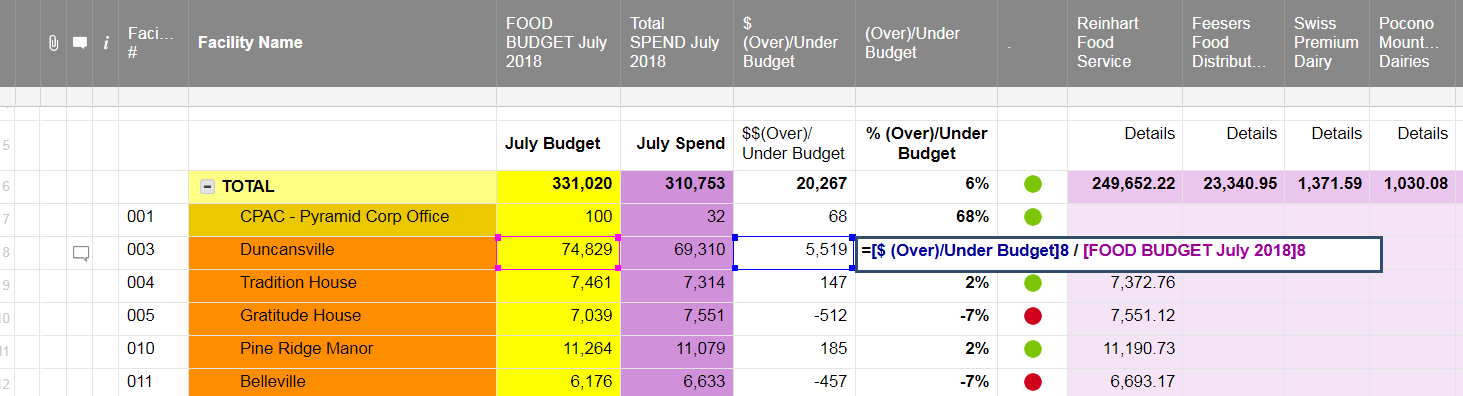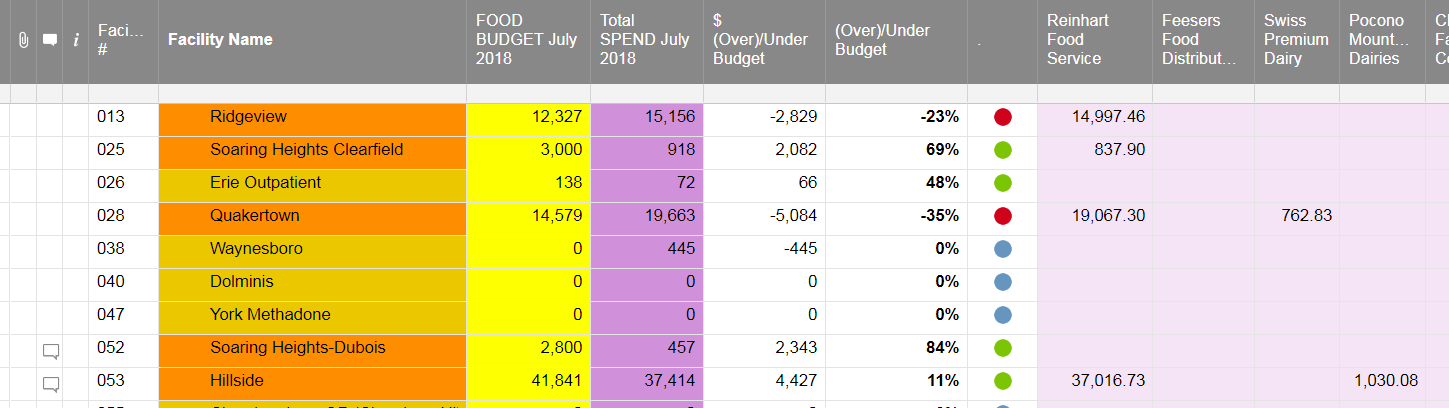Divide by Zero error

08/09/18 Edited 12/09/19

Hello,

I have the following formula to calculate % Over/Under Budget:

=[\$ (Over)/Under Budget]8 / [FOOD BUDGET July 2018]8

I would like this to work even when there is a 0 in the Budget number and even when "over/under" is 0.

ToddTags:

• Either account for 0

=IF([FOOD BUDGET July 2018]@row = 0, 0, [\$ (Over)/Under Budget]@row/ [FOOD BUDGET July 2018]@row)

or catch the error

=IFERROR([\$ (Over)/Under Budget]@row/ [FOOD BUDGET July 2018]@row), 0)

Both of these return 0 if there is a divide by 0.

Craig

• Thanks Craig,

IFERROR works good, now I think my issue has changed.

Now I need to be able handle divide by zero error and if there is a value in "Total Spend..." column against the "Food Budget..." which could be 0.

Added new screenshot to help show - "Waynesboro" line.  Need to be able to show a negative over/under budget even when budget is 0.

Thanks again,

Todd• How do you define a percentage when the budget value is 0?

By "you", I mean you, specifically. Mathematically it has no meaning (is infinite).

What do you want to see as the result of

Budget =0

Spend =445

or Spend = 1 or 1000, as the answer is likely the same.

Personally, I would not return a numerical value, but rather something like "Unexpected Expense!" and the have the RYGB ball be red.

Craig